• 一维切片就不说了，比较简单，先说下二维的，二维的理解了多维的就简单了。举个例子先建立一个5×5的二维数组 多维的切片是按照各个维度分别取 这里就分别输出第一维的2-3和第二维的3-5（索引从0开始）。 这里是...python
• 高维数组子类型,也就是低一维的数组. 子数组类型为array_base,支持array_base所有操作,但是不再支持原来数组特定 操作子数组由[]运算符得到, sa3 da3 //类型均为array_base<;int,2>;...
• ## python 多维切片之冒号和三个点

万次阅读 多人点赞 2017-09-17 15:46:24
一维切片就不说了，比较简单，先说下二维的，二维的理解了多维的就简单了。举个例子先建立一个5x5的二维数组 多维的切片是按照各个维度分别取 比如 这里就分别输出第一维的2-3和第二维的3-5（索引从0开始）。 ...
初学python和numpy，对在学习多维切片的过程中遇到的问题做个总结。
一维切片就不说了，比较简单，先说下二维的，二维的理解了多维的就简单了。举个例子先建立一个5x5的二维数组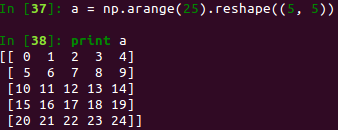多维的切片是按照各个维度分别取
比如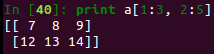这里就分别输出第一维的2-3和第二维的3-5（索引从0开始）。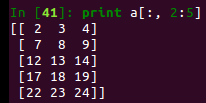这里是行取全部，列取第3-5。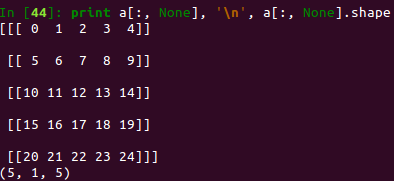这里应该是大家最疑惑的地方了，为什么列的参数改成None，输出的shape都变了，这里大家要知道，None代表新增加一个维度，它有一个别称叫newaxis，大家可以输出一下numpy.newaxis就知道了，那么这个别称应该顾名思义了吧。那么为什么是5x1x5，而不是5x5x1呢，那是因为你在第二维上用了None，你如果在第三维上用就会变成5x5x1了，不信你看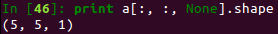这下大家应该明白了吧。就是说None放在哪一维，就会在哪一维上出现新的维度。
再看个更奇葩的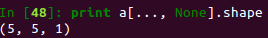三个点是什么鬼，Matlab里面这不是换行的操作么，但这里不是，它是省略所有的冒号来用省略号代替,大家看这个a[:, :, None]和a[…, None]的输出是一样的，就是因为…代替了前面两个冒号。这下应该清楚了。
多维数组和二维的数组可以一样去理解。


展开全文python 索引 numpy
• 多维数组前面讨论都是一维数组，它和二维或多维数组是否相同？它们在某种程度上是相同。然而，指针和数组名称之间差异得更为明显。考虑第5章末尾在井字程序中使用数组。数组声明如下：char board = ...
多维数组
前面讨论的都是一维数组，它和二维或多维数组是否相同？它们在某种程度上是相同的。然而，指针和数组名称之间的差异变得更为明显。考虑第5章末尾在井字程序中使用的数组。数组声明如下：
char board = {
{'1','2','3'},
{'4','5','6'},
{'7','8','9'}
};
本节的例子将使用这个数组，探讨多维数组和指针的关系。
试试看：使用二维数组
这个例子说明了地址和数组board的关系：
/* Program 7.7 Two-Dimensional arrays and pointers */
#include <stdio.h>
int main(void)
{
char board = {
{'1','2','3'},
{'4','5','6'},
{'7','8','9'}
};
printf("address of board         : %p/n", board);
printf("address of board   : %p/n", &board);
printf("but what is in board : %p/n", board);
return 0;
}
输出如下：
address of board          : 0x0013ff67
address of board   : 0x0013ff67
but what is in board   : 0x0013ff67
代码的说明
可以看到，3个输出值都是相同的，从中可以得到什么推论？答案相当简单：声明一维数组时，[n1]放在数组名称之后，告诉编译器它是一个有n1个元素的数组。声明二维数组时，在第一维[n1]的后面放置第二维[n2]，编译器就会创建一个大小为n1的数组，它的每个元素是一个大小为n2的数组。
如第5章所述，声明二维数组时，就是在创建一个数组的数组。因此，用数组名称和一个索引值访问这个二维数组时，例如board，就是在引用一个子数组的地址。仅使用二维数组名称，就是引用该二维数组的开始地址，它也是第一个子数组的开始地址。
总之，board、board和&board 的数值相同，但它们并不是相同的东西。
也就是说，表达式board和board的地址相同。这很容易理解，因为board是第二个子数组board的第一个元素。
但是，用指针记号获取数组中的值时，就会出问题。仍然必须使用间接运算符，但要非常小心。如果改变上面的例子，显示第一个元素的值，就知道原因了：
/* Program 7.7 A Two-Dimensional arrays */
#include <stdio.h>
int main(void)
{
char board = {
{'1','2','3'},
{'4','5','6'},
{'7','8','9'}
};
printf("value of board : %c/n", board);
printf("value of *board :    %c/n", *board);
printf("value of **board :      %c/n", **board);
return 0;
}
这个程序的输出如下：
value of board : 1
value of *board   : 1
value of **board      : 1
可以看到，如果使用board获取第一个元素的值，就需使用两个间接运算符**board。前面的程序可以只使用一个*，是因为那是一维数组。如果只使用一个*，只会得到子数组的第一个元素，即board引用的地址。
多维数组和它的子数组之间的关系如图7-3所示。图7-3  引用数组、其子数组和元素
如图7-3所示，board引用子数组中第一个元素的地址，而board、board和board引用对应子数组中第一个元素的地址。用两个索引值访问存储在数组元素中的值。明白了多维数组是怎么回事，下面看看如何使用board得到数组中的所有值。
试试看：得到二维数组中的所有值
这个例子用for循环进一步改进前一个例子：
/* Program 7.8 Getting the values in a two-dimensional array */
#include <stdio.h>
int main(void)
{
char board = {
{'1','2','3'},
{'4','5','6'},
{'7','8','9'}
};
/* List all elements of the array */
for(int i = 0; i < 9; i++)
printf(" board: %c/n", *(*board + i));
return 0;
}
程序的输出如下：
board:   1
board:   2
board:   3
board:   4
board:   5
board:   6
board:   7
board:   8
board:   9
代码的说明
这个程序要注意在循环中取消引用board的方法：
printf("  board:  %c/n",  *(*board + i));
可以看到，使用表达式*(*board+i)可以得到一个数组元素的值。括号中的表达式*board+i会得到数组中偏移量为i的元素的地址。取消对它的引用，会得到这个地址中的值。括号在这里是很重要的。省略它们会得到board所指向的值(即存储在board中的地址所引用的值)再加上i的值。因此，如果i的值是2，*board+i会得到数组的第一个元素值加2。我们真正想要的是将i的值加到board中的地址，然后对这个新地址取消引用，得到一个值。
下面去掉例子中的括号，看看会发生什么。改变数组的初值，使字符变成从'9'到'1'。如果去掉printf()函数调用中表达式的括号：
printf(" board: %c/n", **board + i);
会得到如下输出：
board: 9
board: :
board: ;
board: <
board: =
board: >
board: ?
board: @
board: A
这是因为i的值加到数组board中的第一个元素上。在ASCII表中，得到的字符是从'9'到'A'。
另外，如果使用表达式**(board+i)，一样会导致错误的结果。此时，**(board+0)指向board，而**(board+1)指向board，**(board+2)指向board。如果增加的数值过大，就会访问数组以外的内存位置，因为这个数组没有第4个元素。
7.3.1  多维数组和指针
前面通过指针的表示法用数组名称引用二维数组，现在学习使用声明为指针的变量。如前所述，这有非常大的区别。如果声明一个指针，给它指定数组的地址，就可以用该指针访问数组的成员。
试试看：多维数组和指针
这个例子使用了多维数组和指针：
/* Program 7.9 Multidimensional arrays and pointers*/
#include <stdio.h>
int main(void)
{
char board = {
{'1','2','3'},
{'4','5','6'},
{'7','8','9'}
};
char *pboard = *board; /* A pointer to char */
for(int i = 0; i < 9; i++)
printf(" board: %c/n", *(pboard + i));
return 0;
}
输出和前一个例子相同：
board:   1
board:   2
board:   3
board:   4
board:   5
board:   6
board:   7
board:   8
board:   9
代码的说明
这里用数组中第一个元素的地址初始化指针，然后用一般的指针算术运算遍历整个数组：
char *pboard = *board;     /* A pointer to char */
for(int i = 0; i < 9; i++)
printf(" board: %c/n", *(pboard + i));
注意，取消了对board的引用(*board)，得到了需要的地址，因为board是数组board的地址，而不是一个元素的地址。可以用以下的方式初始化指针pboard：
char *pboard = &board;
效果相同。用下面的语句初始化指针pboard：
pboard = board;     /* Wrong level of indirection! */
这是错误的。如果这么做，至少会得到编译器的警告。严格地讲，这是不合法的，因为pboard和board有不同的间接级别。这个专业术语的意思是pboard指针引用的地址包含一个char类型的值，而board引用一个地址，那个地址引用另一个含有char类型值的地址。board比pboard多了一级。因此，pboard指针需要一个*，以获得地址中的值，而board需要两个*。一些编译器允许这么用，但是会给出一条警告信息。然而，这是很糟的用法，不应这么用!
7.3.2  访问数组元素
可以使用几种方法访问二维数组的元素。表7-1列出了访问board数组的方法。最左列包含board数组的行索引值，最上面的一行包含列索引值。表中对应于给定行索引和列索引的项列出了引用该元素的各种表达式。
表7-1  访问数组元素的指针表达式

board

0

1

2

0

board
*board
**board

board
*(board+1)
*(*board+1)

board
*(board+2)
*(*board+2)

1

board
*(board+3)
*board
*(*board+3)

board
*(board+4)
*(board+1)
*(*board+4)

board
*(board+5)
*(board+2)
*(*board+5)

2

board
*(board+6)
*(board+3)
*board
*(*board+6)

board
*(board+7)
*(board+4)
*(board+1)
*(*board+7)

board
*(board+8)
*(board+5)
*(board+2)
*(*board+8)

下面看看如何把前面所学的指针知识应用于前面没有使用指针编写的程序中，然后就可以看出基于指针的实现方式有什么不同了。第5章编写了一个计算帽子尺寸的例子，下面用另一种方式完成这个例子。
试试看：帽子尺寸的另一种计算方法
使用指针表示法重写帽子尺寸的例子：
/* Program 7.10 Understand pointers to your hat size - if you dare */
#include <stdio.h>
#include <stdbool.h>
int main(void)
{
char size = {                      /* Hat sizes as characters */
{'6', '6', '6', '6', '7', '7', '7', '7', '7', '7', '7', '7'},
{'1', '5', '3', '7', ' ', '1', '1', '3', '1', '5', '3', '7'},
{'2', '8', '4', '8', ' ', '8', '4', '8', '2', '8', '4', '8'}
};
int headsize =                            /* Values in 1/8 inches */
{164,166,169,172,175,178,181,184,188,191,194,197};
char *psize = *size;
int *pheadsize = headsize;
float cranium = 0.0;     /* Head circumference in decimal inches */
int your_head = 0;       /* Headsize in whole eighths */
bool hat_found = false; /* Indicates when a hat is found to fit */
bool too_small = false; /* Indicates headsize is too small */
/* Get the circumference of the head */
printf("/nEnter the circumference of your head above your eyebrows"
" in inches as a decimal value: ");
scanf(" %f", &cranium);
/* Convert to whole eighths of an inch */
your_head = (int)(8.0*cranium);
/* Search for a hat size */
for(int i = 0 ; i < 12 ; i++)
{
/* Find head size in the headsize array */
if(your_head > *(pheadsize+i))
continue;
/* If it is the first element and the head size is */
/* more than 1/8 smaller then the head is too small */
/* for a hat */
if((i == 0) && (your_head < (*pheadsize)-1))
{
printf("/nYou are the proverbial pinhead. No hat for"
"you I'm afraid./n");
too_small = true;
break;           /* Exit the loop */
}
/* If head size is more than 1/8 smaller than the current */
/* element in headsize array, take the next element down */
/* as the head size */
if( your_head < *(pheadsize+i)-1)
i--;
printf("/nYour hat size is %c %c%c%c/n",
*(psize + i),         /* First row of size */
*(psize + 1*12 + i), /* Second row of size */
(i==4) ?' ' : '/',
*(psize+2*12+i));     /* Third row of size */
hat_found=true;
break;
}
if(!hat_found && !too_small)
printf("/nYou, in technical parlance, are a fathead."
" No hat for you, I'm afraid./n");
return 0;
}
这个程序的输出和第5章相同，所以不再重复。这里关心的是代码本身，下面看看这个程序的新元素。
代码的说明
这个程序的执行过程和第5章相同。其区别是这个实现代码使用了指针pheadsize和psize，它们分别包含headsize数组和size数组的开始地址。your_head的值和数组的值用下面的语句作比较：
if(your_head > *(pheadsize+i))
continue;
比较运算符右侧的表达式*(pheadsize+i)等于数组表示法中的headsize[i]。括号内的表达式把i加到数组开始的地址上。给地址加一个整数值，会给该地址加上元素长度的i倍值。因此，括号内的子表达式会产生对应于索引值为i的元素的地址。然后，使用取消引用运算符*，得到这个元素的内容，将它和变量your_head的值进行比较。
如果在中间执行printf ()，可以看到访问某行一个元素的指针表达式对二维数组的执行结果：
printf("/nYour hat size is %c %c%c%c/n",
*(psize + i),          /* First row of size */
*(psize + 1*12 + i), /* Second row of size */
(i==4) ?' ' : '/',
*(psize+2*12+i));     /* Third row of size */
第一个表达式是*(psize+i)，它访问size数组中第一行的第i个元素，等于size[i]。第二个表达式是*(psize + 1*12 + i)，它访问size数组中第二行的第i个元素，等于size[i]。这个表达式说明了第二行的开始位置可以通过给psize加上行的大小来得到。接着给该结果加上i，就得到了第二行中的元素。要得到size数组中第三行的元素，可以使用表达式*(psize+2*12+i)，它等于size[i]。
展开全文arrays 编译器 存储 search
• 高中时候我们便学过一维正态（高斯）分布公式： 拓展到高维时，就变成： 其中， 表示维度为 D 向量， 则是这些向量平均值，Σ 表示所有向量 协方差矩阵。 本文只是想简单探讨一下，上面这个高维...
高中的时候我们便学过一维正态（高斯）分布的公式：

拓展到高维时，就变成：

其中， 表示维度为 D 的向量， 则是这些向量的平均值，Σ 表示所有向量  的协方差矩阵。

本文只是想简单探讨一下，上面这个高维的公式是怎么来的。

二维的情况

为了简单起见，本文假设所有变量都是相互独立的。即对于概率分布函数  而言，有  成立。

现在，我们用一个二维的例子推出上面的公式。

假设有很多变量  ，它们的均值为  ，方差为  。

由于 𝑥1，𝑥2 是相互独立的，所以， 的高斯分布函数可以表示为：

接下来，为了推出文章开篇的高维公式，我们要想办法得到协方差矩阵 Σ 。

对于二维的向量  而言，其协方差矩阵为：

(不熟悉协方差矩阵的请查找其他资料或翻看我之前的文章)

由于 𝑥1，𝑥2 是相互独立的，所以  。这样，Σ 退化成  。

则 Σ 的行列式  ，代入公式 (4) 就可以得到：

这样一来，我们已经推出了公式的左半部分，下面，开始处理右面的 𝑒𝑥𝑝函数。

原始的高维高斯函数的 𝑒𝑥𝑝 函数为：，根据前面算出来的 Σ，我们可以求出它的逆： 。

接下来根据这个二维的例子，将原始的 𝑒𝑥𝑝()展开：

展开到最后，发现推出了公式 (4)。说明原公式  是成立的。你也可以将上面展开的过程逆着推回去，一样可以从例子中的公式 (4) 推出多维高斯公式。

函数图像

知道多维的公式后，下面再简单比较一下一维和二维的图像区别。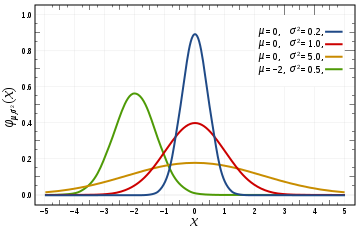上图展示的是 4 个一维高斯函数的图像。高斯函数是一个对称的山峰状，山峰的中心是均值 𝑢 ，山峰的「胖瘦」由标准差 𝜎 决定，如果 𝜎 越大，证明数据分布越广，那么山峰越「矮胖」，反之，则数据分布比较集中，因此很大比例的数据集中在均值附近，山峰越「瘦高」。在偏离均值 𝑢 三个 𝜎 的范围外，数据出现的概率几乎接近 0，因此这一部分的函数图像几乎与 x 轴重合。下面看二维的例子：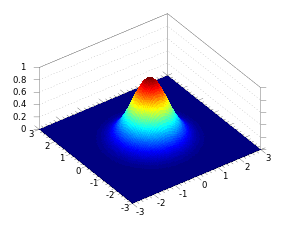有了一维图像的例子，二维图像就可以类比出来了。如果说，一维只是山峰的一个横截面，那么二维则是一个完整的有立体感的山峰。山峰的「中心」和「胖瘦」和一维的情况是一致的，而且，对于偏离中心较远的位置，数据出现的概率几乎为 0，因此，函数图像在这些地方就逐渐退化成「平原」了。

参数估计

另外，如果给定了很多数据点，并且知道它们服从某个高斯分布，我们要如何求出高斯分布的参数（𝜇和 Σ）呢？

当然，估计模型参数的方法有很多，最常用的就是极大似然估计。

简单起见，拿一维的高斯模型举例。假设我们有很多数据点： ，它们的均值是𝑢̃ 。一维高斯函数是

首先，我们先写出似然函数：

然后取对数：

求出导数，令导数为 0 得到似然方程：

我们可以求出： ，可以看到，这其实就是高斯函数中平均值和标准差的定义。

对于高维的情况，平均值和协方差矩阵也可以用类似的方法计算出来。

总结

本文只是从一个简单的二维例子出发，来说明多维高斯公式的来源。在 PRML 的书中，推导的过程更加全面，也复杂了许多，想深入学习多维高斯模型的还是参考教材为准。

重新对比一维和多维的公式：

其实二者是等价的。一维中，我们针对的是一个数，多维时，则是针对一个个向量求分布。如果向量退化成一维，则多维公式中的  ，这时多维公式就退化成一维的公式。所以，在多维的公式中，我们可以把 Σ 当作是样本向量的标准差。

参考

协方差矩阵
Gaussian function

展开全文• ## MDS多维缩放详解

千次阅读 2019-05-11 12:02:12
开始先上两幅图是因为通过上图我们可以对降维有个直观形象的了解。降维亦称“数约简”，是经过某种数学变换将原始高维属性空间变为个低“子空间”。...上图给出了个直观的例子，原是高维空间中的样本...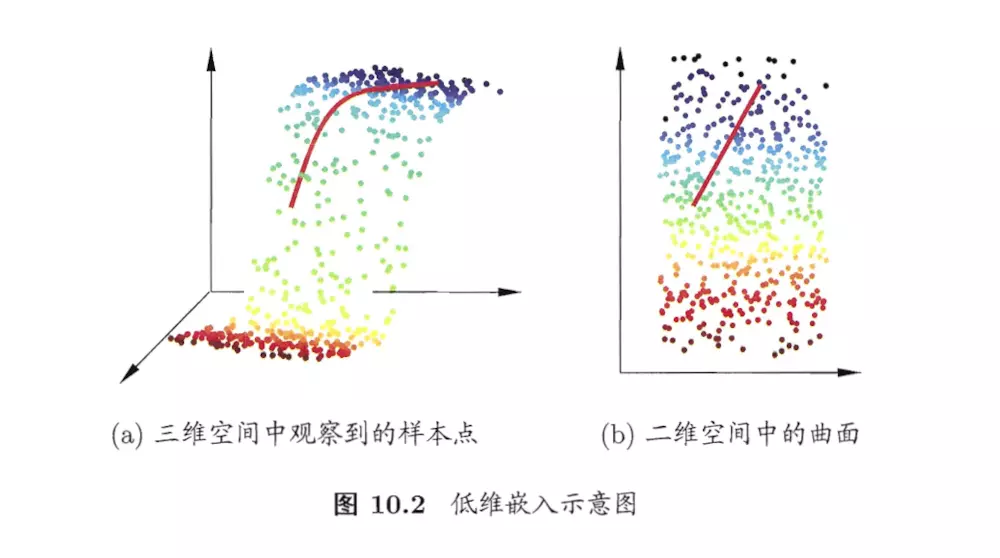一开始先上两幅图是因为通过上图我们可以对降维有一个直观形象的了解。降维亦称“维数约简”，是经过某种数学变换将原始高维属性空间变为一个低维“子空间”。在这个子空间中样本密度大幅度提高，距离计算也变得更为容易。为什么能进行降维？因为在很多时候，人们观测或收集到的数据样本虽是高维的，但是与学习任务密切相关的也许仅是某个低维分布，即高维空间中的一个低维嵌入。上图给出了一个直观的例子，原是高维空间中的样本点，在这个低维嵌入子空间中更容易进行学习。
这篇我们讲的是MDS（Mutiple Dimensional Scaling）多维缩放这样一种经典的降维方法，该方法的核心便是要求原始空间中样本之间的距离在低维空间中得以保持。怎么理解这句话呢，举个简单的例子：就比如说我们要画一张地图，现实中的地球是三维立体的，那么计算现实中个位置间的距离需在三维坐标下进行，但是现在我们要画一张二维的平面地图，当然我们保持其二维坐标中各个位置间的距离要和在三维坐标系下的一致，这就是高维距离与低维距离的一致性。接下的公式推导会让我们对该方法的核心有更深刻的理解。
我们先假定一个样本数量为n，特征维数为m的样本空间$D \in R^{n \times m}$，这n个样本在原始空间中的距离矩阵为$D i s t \in R^{n \times n}$，其中第i行j列的元素$d i s t_{i j}$为样本xi到xj的距离。我们的目标是获得样本在d’维空间的表示$Z \in R^{n \times d^{\prime}}$，d’$\leq$d，且任意两个样本在d’空间中的欧式距离等于原始空间中的距离。那么，我们设定等式:
$\left\|z_{i}-z_{j}\right\|=d i s t_{i j}$在此基础上求得降维后的样本空间Z。
现在我们已知的信息或者说是整个MDS算法的输入是原始的距离矩阵Dist，约束条件是$\left\|z_{i}-z_{j}\right\|=d i s t_{i j}$，接下要做的就是如何通过已知输入和约束条件求得Z。
首先，我们计算一个降维后样本的内积矩阵B，令$B=Z Z^{T} \in R^{n \times n}$，B中每个元素的值为：$b_{i j}=\sum_{k=1}^{d'} z_{i k} z_{j k}$接着我们计算一个降维后关于样本空间的距离矩阵D：
\begin{aligned} d_{i j}^{2} &=\left(z_{i}-z_{j}\right)^{2} \\ &=\sum_{k=1}^{d'}\left(z_{i k}-z_{j k}\right)^{2} \\ &=\sum_{k=1}^{d'} z_{i k}^{2}+z_{j k}^{2}-2 z_{i k} z_{j k} \\ &=b_{i i}+b_{j j}-2 b_{i j} （１） \end{aligned}而根据我们的约束条件，有$d_{ij}^{2}=dist_{ij}^{2} （２）$
为了便于讨论，令降维后的样本Z被中心化，即$\sum_{i=1}^{n} z_{i}=0$。显然，矩阵B的行与列之和均为零，即\begin{aligned} \sum_{i=1}^{n} b_{i j} &=\sum_{i=1}^{n} \sum_{k=1}^{d'} z_{i k} z_{j k} =0 \end{aligned}\begin{aligned} \sum_{j=1}^{n} b_{i j} &=\sum_{j=1}^{n} \sum_{k=1}^{d'} z_{i k} z_{j k} =0 \end{aligned}易知：\begin{aligned} \sum_{i=1}^{n} dist_{i j}^{2} &=\sum_{i=1}^{n} b_{i i}+b_{j j}-2 b_{i j} =tr(B)+n b_{j j}+0 \end{aligned}\begin{aligned} \sum_{j=1}^{n} dist_{i j}^{2} &=\sum_{j=1}^{n} b_{j j}+b_{i i}-2 b_{i j} =tr(B)+n b_{i i}+0 \end{aligned}$\sum_{i=1}^{n} \sum_{j=1}^{n} d i s t^{2}=2 n t r(B)$其中，tr(B)为内积矩阵B的迹，也就是矩阵主对角线元素的和。根据以上公式我们又可以得出：\begin{aligned} b_{i i} &=-\frac{tr(B)}{n}+\frac{1}{n} \sum_{j=1}^{n} dist_{i j}^{2} （３）\\ b_{j j} &=-\frac{tr(B)}{n}+\frac{1}{n} \sum_{i=1}^{n} dist_{i j}^{2} （４） \\ \frac{tr(B)}{n} &=\frac{1}{n^{2}} \sum_{i=1}^{n} \sum_{j=1}^{n} dist_{i j}^{2} （５）\end{aligned} 由式（1）（2）及式（3）~（5）可得：\begin{aligned} b_{i j} &=-\frac{1}{2}\left(dist_{i j}^{2}-b_{i i}-b_{j j}\right) \\ &=-\frac{1}{2}\left(dist_{i j}^{2}-\frac{1}{n} \sum_{j=1}^{n} dist_{i j}^{2}-\frac{1}{n} \sum_{i=1}^{n} dist_{i j}^{2}+\frac{2tr(B)}{n}\right) \\ &=-\frac{1}{2}\left(dist_{i j}^{2}-\frac{1}{n} \sum_{j=1}^{n} dist_{i j}^{2}-\frac{1}{n} \sum_{i=1}^{n} dist_{i j}^{2}+\frac{1}{n^{2}} \sum_{i=1}^{n} \sum_{j=1}^{n} dist_{i j}^{2}\right) \\ &=-\frac{1}{2}\left(dist_{i j}^{2}-dist_{i .}^{2}-dist_{i j}^{2}+dist_{..}^{2}\right) \end{aligned}这样我们通过原始样本空间的距离矩阵Dist求得内积矩阵B。
B是对称矩阵，所以可对矩阵B做特征值分解，$B=V \Lambda V^{T}$，其中$\Lambda=diag(\lambda_{1}, \lambda_{2}, \cdots, \lambda_{m})$为特征值构成的对角矩阵，$\lambda_{1} \geq \lambda_{2} \geq \cdots \geq \lambda_{m}$，V为特征向量矩阵。如果$\Lambda$中恰巧有$m^{*}$个非零的特征值，他们构成对角矩阵$\Lambda_{*}=diag(\lambda_{1}, \lambda_{2}, \cdots, \lambda_{m^{*}})$，令$V_{*}$表示相应的特征向量矩阵，则有：${B^{*}=V^{*} \Lambda^{*} V^{*^{T}}} (V^{*} \in R^{n \times m^{*}}，\Lambda^{*} \in R^{m \times m^{*}})$所以有：\begin{aligned} B^{*} &=V^{*} \Lambda^{* \frac{1}{2}} * \Lambda^{* \frac{1}{2}} V^{* T} \\ &=ZZ^{T} \end{aligned}因此：$Z=V^{*} \Lambda^{* \frac{1}{2}}$若$m^{*}=m$，此时得到的$Z$就是原数据去中心化并做了某种正交变换后的值了（在某个博客中看到说因为D是对称矩阵，此时得到的B刚好会有m个非0的特征值，但是我无法理解这一句，主要也是线性代数没学好，如果有人能验证这句话是真的，欢迎补充）。
原始数据的特征维度为m，我们的最终目的是为了降维，此时可取d’<<m个最大特征值构成的对角矩阵$\tilde{\Lambda}=diag(\lambda_{1}, \lambda_{2}, \cdots, \lambda_{d'})$,，令$\tilde{V}$表示相应的特征向量矩阵，则$Z$的表达式为：$Z=\tilde{\Lambda}^{\frac{1}{2}} \tilde{V}^{T}$Z就是降维后最终得到的低维样本空间矩阵，且Z的距离矩阵和原始的距离矩阵是近似相等的。
讨论
如果我们计算得到的特征值个数等于m，是不是就可以还原到原始的样本空间矩阵呢，答案是肯定的，不可能。为什么呢？回到我们一开始举的那个例子，画一张二维的地图，而如果我们仅仅是要求各位置距离不变的话 ，其实会有很多种结果，因为在三维空间中对各位置进行翻转平移并不会影响位置间的距离，所以在将三维空间降到二维后，我们并不知道对其进行了怎样的转换。MDS的输入是距离矩阵，其实我们通过样本空间计算距离的时候就已经丢失很多信息，但这并不会影响进行有效的数据降维。
思考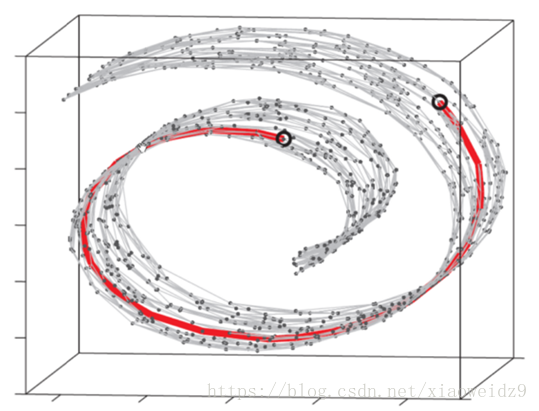以上的图形是Swiss Roll，也就是瑞士卷，对于这样的流形空间，我们用MDS方法是否合适呢，就像上图的两个黑圆圈之间的距离，真实距离是红色的测地线，但是如果我们使用MDS方法，就会直接粗暴地计算两圆圈之间的欧氏距离，可以想象，与真实距离还是有很大差距的，这时就会引出另一种方法叫Isomap等度量映射，之后我可能会再写一篇博客讲讲，其实他的核心还是MDS，只是进行了优化。
算法描述
来自于西瓜书：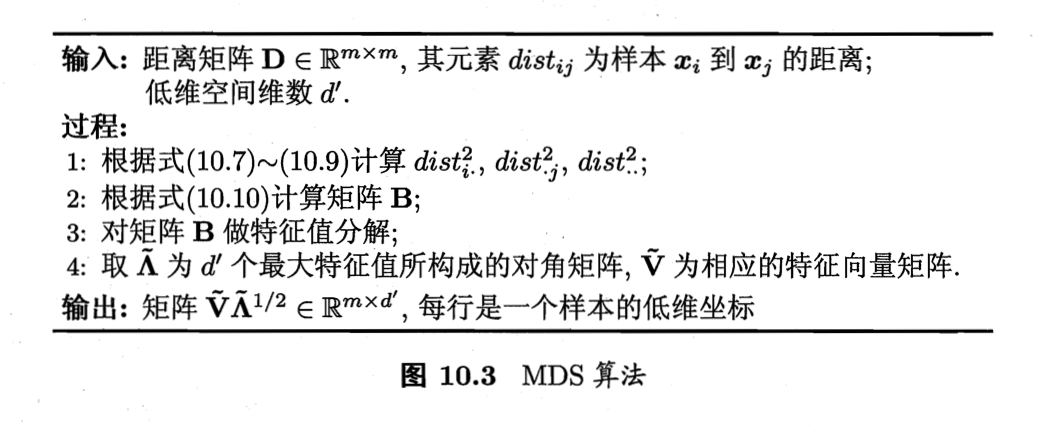接下来有一个简单的算法代码：（代码来源：http://www.cnblogs.com/lochan/p/6627511.html）
import numpy as np

# run this to get a test matrix
# A = np.random.randint(1,100,(5,20))
# np.save('mat.npy', A)
# exit()

A = np.load('mat.npy')

n,m = A.shape
Dist = np.zeros((n,n))
B = np.zeros((n,n))
for i in range(n):
for j in range(n):
Dist[i][j] = sum((ix-jx)**2 for ix,jx in zip(A[i], A[j]))

disti2 = np.array(*n)
distj2 = np.array(*n)

for x in range(n):
disti2[x] = np.mean([Dist[x][j] for j in range(n)])
distj2[x] = np.mean([Dist[i][x] for i in range(n)])

distij2 = np.mean([Dist[i][j] for i in range(n) for j in range(n)])

for i in range(n):
for j in range(n):
B[i][j] = -0.5*(Dist[i][j] - disti2[i] - distj2[j] + distij2)

w,v = np.linalg.eig(B)

v=v.transpose()

U = [{'eVal':w[i], 'eVec':v[i]} for i in range(n)]

U.sort(key = lambda obj:obj.get('eVal'), reverse = True)
k=4
w=np.array(*k)
v=np.zeros((k,n))

for i in range(k):
w[i] = U[i].get('eVal')**0.5
v[i] = U[i].get('eVec')

ans = np.dot(v.transpose(), np.diag(w))

ans_dist = np.zeros((n,n))
for i in range(n):
ans_str=""
for j in range(n):
ans_dist[i][j] = sum((ix-jx)**2 for ix,jx in zip(ans[i], ans[j]))

print("Orign dis[][] is :")
print Dist
print("MDS dis[][] is :")
print(ans_dist)

将算法和代码结合起来看，大家应该会对MDS的整个过程有更加清楚的了解。
注：
不同的书和博客上建立的原始样本空间矩阵是不一样的，有的是矩阵的一行代表一个样本，有的是一列，这对公式的推导过程有很大影响，大家要注意，很容易混淆。
参考文献：
1、周志华《机器学习》
2、http://www.cnblogs.com/lochan/p/6627511.html
3、https://blog.csdn.net/Dark_Scope/article/details/53229427


展开全文MDS 机器学习
• 个强大N数组对象Array； 比较成熟（广播）函数库； 用于整合C/C++和Fortran代码工具包； 实用线性代数、傅里叶变换和随机数生成函数。 SciPy是个开源Python算法库和数学工具包，SciPy包含...
• 我们在使用多维数组是有一点，任何情况下只能省略第一维的长度。比如在函数中要传一个数组时，数组行可以在函数调用时传递，当属数组列却只能在能被预置在函数内部。看下面一个例子： #define COLS 4 int ...
• 9.1.4 一维数组clone()方法 212 9.1.5 当数组类型不再是基本数据类型 214 9.1.6 多维数组clone()方法 217 9.2 老朋友String类 220 9.2.1 遍历String中字符 220 9.2.2 获取字符串中一部分 222 9.2.3 ...JAVA 入门
• 9.1.4 一维数组clone()方法 212 9.1.5 当数组类型不再是基本数据类型 214 9.1.6 多维数组clone()方法 217 9.2 老朋友String类 220 9.2.1 遍历String中字符 220 9.2.2 获取字符串中一部分 222 9.2.3 ...JAVA 入门
• 9.1.4 一维数组clone()方法 212 9.1.5 当数组类型不再是基本数据类型 214 9.1.6 多维数组clone()方法 217 9.2 老朋友String类 220 9.2.1 遍历String中字符 220 9.2.2 获取字符串中一部分 222 9.2.3 ...JAVA 入门
• ImgLib2是通用的多维图像处理库。 它提供了种接口驱动的设计，该设计以可扩展的方式支持数字... 例如，我们有一些研究RNA序列的例子。 ImgLib2提供透明的数据访问。 该算法不需要知道它正在虚拟堆栈上运行，并且实Java
• 文章目录ndarray数组对象维度操作视图变维(数据共享)复制变维（数据独立）就地变维ndarray数组切片操作多维数组切片操作ndarray数组掩码操作多维数组组合和拆分垂直方向操作水平方向操作深度方向操作(3)...python numpy 数据分析
• 如果你已经知晓 C++ 语言，本文将在不到小时时间内带你快速浏览 C# 语法。如果熟悉 Java 语言，Java 编程结构、打包和垃圾回收概念肯定对你快速学习 C# 大有帮助。所以我在讨论 C# 语言构造时候会假设你...
• 3.1 一维小波变换 Matlab 实现 (1) dwt 函数 功能：一维离散小波变换 格式：[cA,cD]=dwt(X,'wname') [cA,cD]=dwt(X,Lo_D,Hi_D) 说明：[cA,cD]=dwt(X,'wname') 使用指定小波基函数 'wname' 对信号 X 进行分解，...
• 数组可以是一维的，也可是多维的。数祖的成员可以是整齐的，也可以是长（jagged）的。 一维的数组是最普通，最简单的。这里值给出一个例子，就不多解释了。*/ using System; class Test { static void Main() { ...c# class system
• ## 你必须知道的495个C语言问题

千次下载 热门讨论 2015-05-08 11:09:25
6.18　当我向个接受指针指针函数传入二数组时候，编译器报错了。 6.19　我怎样编写接受编译时宽度未知数组函数？ 6.20　我怎样在函数参数传递时混用静态和动态多维数组？ 数组大小 6.21　当...C语言
• 文章目录例子基本概念简单泛型使用Object使用泛型元组(tuple)二元组多维元组简单使用元组泛型方法泛型方法定义可参数+泛型方法 例子 天，小吴和小范两个程序员来到了相亲会所找对象，相亲会所里面美女让人...java
• 5 数组类型（Array types） 数组可以是一维的，也可是多维的。数祖的成员可以是整齐的，也可以是长（jagged）的。 一维的数组是最普通，最简单的。这里值给出一个例子，就不多解释了。*/ using System; class Test...c# class system
• 5、5系数二阶椭圆方程Dirichlet问题Chebyshev拟谱方法 5、6定常Burgers方程Chebyshev谱方法 参考文献 第六章 谱方法某些新进展 6、1用Gegenbauer多项式恢复指数精度 6、1、1Gegenbauer多项式及其主要性质 6...
• 可我找不到任何方法来声明这样函数——感觉我需要个返回指针函数，返回指针指向又是返回指针函数……，如此往复，以至无穷。　12　 数组大小　13 1.23　能否声明和传入数组大小一致局部数组，或者由...C 进阶
• 可我找不到任何方法来声明这样函数——感觉我需要个返回指针函数，返回指针指向又是返回指针函数……，如此往复，以至无穷。　12　 数组大小　13 1.23　能否声明和传入数组大小一致局部数组，或者由...